AP State Board Syllabus AP SSC 10th Class Maths Textbook Solutions Chapter 12 Applications of Trigonometry Ex 12.2 Textbook Questions and Answers.

## AP State Syllabus SSC 10th Class Maths Solutions 12th Lesson Applications of Trigonometry Exercise 12.2

### 10th Class Maths 12th Lesson Applications of Trigonometry Ex 12.2 Textbook Questions and Answers

Question 1.
A TV tower stands vertically on the side of a road. From a point on the other side directly opposite to the tower, the angle of elevation of the top of tower is 60°. From another point 10 m away from this point, on the line joining this point to the foot of the tower, the angle of elevation of the top of the tower is 30°. Find the height of the tower and the width of the road.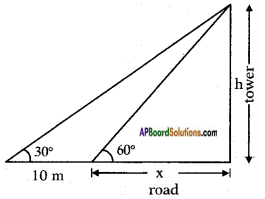Let the height of the tower = h mts say
Width of the road be = x m.
Distance between two points of observation = 10 cm.
Angles of elevation from the two points = 60° and 30°.
From the figure
tan 60° = $$\frac{h}{x}$$
√3 = $$\frac{h}{x}$$
⇒ h = √3x …….(1)
Also tan 30° = $$\frac{h}{10+x}$$
⇒ $$\frac{1}{\sqrt{3}}$$ = $$\frac{h}{10+x}$$
⇒ h = $$\frac{10+x}{\sqrt{3}}$$ ………(2)
From equations (1) and (2) h
h = √3x = $$\frac{10+x}{\sqrt{3}}$$
∴ √3x = $$\frac{10+x}{\sqrt{3}}$$
√3 × √3x = 10 + x
⇒ 3x – x = 10
⇒ 2x = 10
⇒ x = $$\frac{10}{2}$$ = 5m
∴ Width of the road = 5 m
Now Height of the tower = √3x = 5√3 m.Question 2.
A 1.5 m tall boy is looking at the top of a temple which is 30 metre in height from a point at certain distance. The angle of elevation from his eye to the top of the crown of the temple increases from 30° to 60° as he walks towards the temple. Find the distance he walked towards the temple.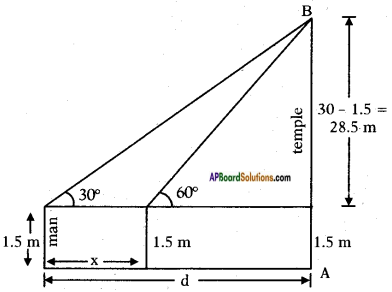Height of the temple = 30 m
Height of the man = 1.5 m
Initial distance between the man and temple = d m. say
Let the distance walked = x m.
From the figure
tan 30° = $$\frac{30-1.5}{d}$$
⇒ $$\frac{1}{\sqrt{3}}$$ = $$\frac{28.5}{d}$$
∴ d = 28.5 × √3m ………(1)
Also tan 60° = $$\frac{28.5}{d-x}$$
⇒ √3 = $$\frac{28.5}{d-x}$$
⇒ √3(d-x) = 28.5
⇒ √3(28.5 × √3-x) = 28.5
⇒ 28.5 × 3 – √3x = 28.5
⇒ √3x = 3 × 28.5-28.5
⇒ √3x = 2 × 28.5 = 57
∴ x = $$\frac{57}{\sqrt{3}}=\frac{19 \times 3}{\sqrt{3}}$$ = 19√3
= 19 × 1.732
= 32.908 m.
∴ Distance walked = 32.908 m.

Question 3.
A statue stands on the top of a 2 m tall pedestal. From a point on the ground, the angle of elevation of the top of the statue is 60° and from the same point, the angle of elevation of the top of the pedestal is 45°. Find the height of the statue.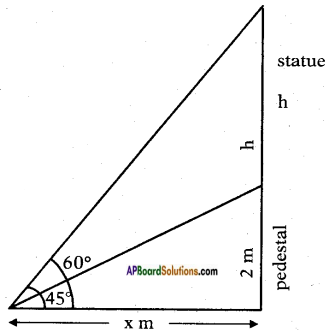Height of the pedestal = 2 m.
Let the height of the statue = h m. Angle of elevation of top of the statue = 60°.
Angle of elevation of top of the pedestal = 45°.
Let the distance between the point of observation and foot of the pedestal = x m.
From the figure
tan 45° = $$\frac{2}{x}$$
1 = $$\frac{2}{x}$$
∴ x = 2 m.
Also tan 60° = $$\frac{2+h}{x}$$
⇒ √3 = $$\frac{2+h}{x}$$
⇒ 2√3 = 2 + h
⇒ h = 2√3 – 2
= 2(√3-1)
= 2(1.732 – 1)
= 2 × 0.732
= 1.464 m.Question 4.
From the top of a building, the angle of elevation of the top of a cell tower is 60° and the angle of depression to its foot is 45°. If distance of the building from the tower is 7 m, then find the height of the tower.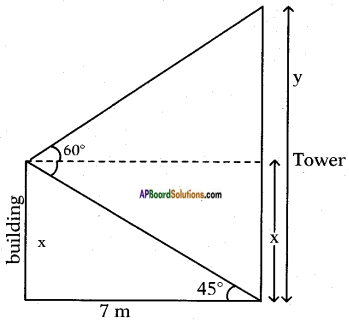Angle of elevation of the top of the tower = 60°.
Angle of depression to the foot of the tower = 45°.
Distance between tower and building = 7 m.
Let the height of the building = x m and tower = y m.
From the figure
tan 45° = $$\frac{x}{7}$$
1 = $$\frac{x}{7}$$
∴ x = 7 m.
Also tan 60° = $$\frac{y-x}{7}$$
⇒ √3 = $$\frac{y-x}{7}$$
⇒ 7√3 = y – 7
∴ y = 7 + 7√3
= 7 (√3 + 1)
= 7(1.732 + 1)
= 2.732 × 7
= 19.124 m.

Question 5.
A wire of length 18 m had been tied with electric pole at an angle of eleva¬tion 30° with the ground. As it is covering a long distance, it was cut and tied at an angle of elevation 60° with the ground. How much length of the wire was cut ?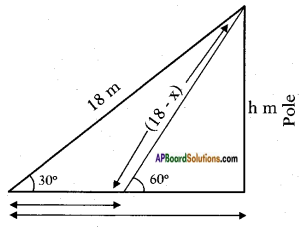Length of the wire = 18 m
Let the length of the wire removed = x
Height of the pole be = h
From the figure
sin 30° = $$\frac{h}{18}$$
⇒ $$\frac{1}{2}$$ = $$\frac{h}{18}$$
⇒ h = $$\frac{18}{2}$$ = 9 m
Also sin 60° = $$\frac{h}{18-x}$$
$$\frac{\sqrt{3}}{2}$$ = $$\frac{9}{18-x}$$
√3(18-x) = 9 × 2
18√3 – √3x = 18
√3x = 18√3 – 18
√3x = 18(√3-1)
x = $$\frac{18(\sqrt{3}-1)}{\sqrt{3}}$$
= $$\frac{6 \times 3(\sqrt{3}-1)}{\sqrt{3}}$$
= 6√3(√3-1)
= 6(3-√3)
= 18 – 6√3
= 18 – 10.392
= 7.608 m.Question 6.
The angle of elevation of the top of a building from the foot of the tower is 30° and the angle of elevation of the top of the tower from the foot of the building is 60°. If the tower is 30 m high, find the height of the building.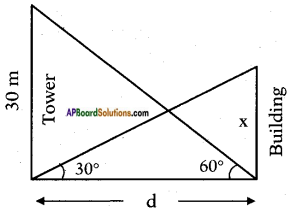Height of the tower = 30 m
Angle of elevation of the top of the tower = 60°.
Angle of elevation of the top of the building = 30°.
Let the distance between the foot of the tower and foot of the building be d m and height of the building be x m.
From the figure
tan 60° = $$\frac{30}{d}$$
√3 = $$\frac{30}{d}$$
⇒ d = $$\frac{30}{\sqrt{3}}=\frac{10 \times 3}{\sqrt{3}}$$ = 10√3m
Also tan 30° = $$\frac{x}{d}$$
⇒ $$\frac{1}{\sqrt{3}}=\frac{x}{10 \sqrt{3}}$$
⇒ x = $$\frac{10 \sqrt{3}}{\sqrt{3}}$$ = 10 m
∴ Height of the building = 10 m

Question 7.
Two poles of equal heights are standing opposite to each other on either side of the road, which is 120 feet wide. From a point between them on the road, the angles of elevation of the top of the poles are 60° and 30° respectively. Find the height of the poles and the distances of the point from the poles.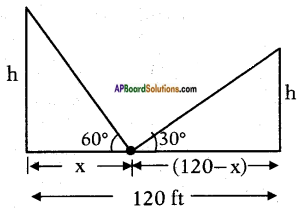Width of the road = 120 f.
Angle of elevation of the top of the 1st tower = 60°.
Angle of elevation of the top of the 2 tower = 30°.
Let the distance of the point from the 1st pole = x.
Then the distance of the point from
the 2nd pole = 120 – x.
and height of each pole = h say.
From the figure
tan 60° = $$\frac{h}{x}$$
⇒ √3 = $$\frac{h}{x}$$
⇒ h = √3x ……..(1)
Also tan 30° = $$\frac{\mathrm{h}}{120-\mathrm{x}}$$
⇒ $$\frac{1}{\sqrt{3}}=\frac{\mathrm{h}}{120-\mathrm{x}}$$
⇒ h = $$\frac{120-x}{\sqrt{3}}$$
From (1) and (2)
√3x = $$\frac{120-x}{\sqrt{3}}$$
⇒ √3.√3x = 120-x
⇒ 3x = 120 – x
⇒ 3x + x = 120
⇒ 4x = 120
⇒ x = $$\frac{120}{4}$$ = 30 ft
Now h = √3x = √3 × 30 = 1.732 x 30 = 51.960 feet
∴ Distances of the poles = 30 ft. and 120 – 30 fts = 90 ft.
Height of each pole = 51.96 ft.Question 8.
The angles of elevation of the top of a tower from two points at a distance of 4 m and 9 m, find the height of the tower from the base of the tower and in the same straight line with it are complementary.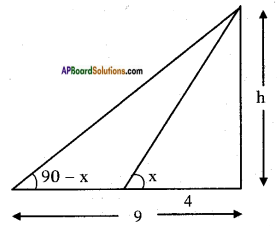Let the height of the tower = h m.
Angles of elevation of the top of the tower from two points = x° and (90° – x)
From the figure
tan x = $$\frac{h}{4}$$ ……. (1)
Also tan (90° – x) = $$\frac{h}{9}$$
⇒ cot x = $$\frac{h}{9}$$
⇒ $$\frac{1}{\tan x}$$ = $$\frac{h}{9}$$
∴ tan x = $$\frac{9}{h}$$ …….. (2)
From (1) and (2)
tan x = $$\frac{h}{4}$$ = $$\frac{9}{h}$$
∴ $$\frac{h}{4}$$ = $$\frac{9}{h}$$
h × h = 9 × 4
⇒ h2 = 36
⇒ h = 6 m

Question 9.
The angle of elevation of a jet plane from a point A on the ground is 60°. After 4 flight of 15 seconds, the angle of elevation changes to 30°. If the jet plane is flying at a constant height of 1500√3 meter, find the speed of the jet plane. (√3 = 1.732)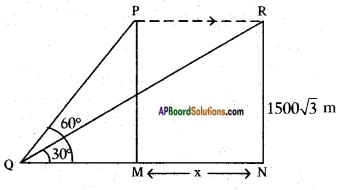Height of the plane from the ground PM = RN = 1500√3 m.
Angle of elevation are 30° and 60°.
From the figure
tan 60° = $$\frac{PM}{QM}$$
√3 = $$\frac{1500 \sqrt{3}}{\mathrm{QM}}$$
QM = $$\frac{1500 \sqrt{3}}{\sqrt{3}}$$ = 1500 m
Also tan 30° = $$\frac{RN}{QN}$$
$$\frac{1}{\sqrt{3}}=\frac{1500 \sqrt{3}}{\mathrm{QM}+\mathrm{MN}}$$
QM + MN = 1500√3 × √3
1500 + MN = 1500 × 3
MN = 4500 – 1500
MN = 3000 mts.
∴ Distance travelled in 15 seconds = 3000 mts.
∴ Speed of the jet plane = $$\frac{\text { distance }}{\text { time }}=\frac{3000}{15}$$ = 200 m/s
= 200 × $$\frac{18}{5}$$ kmph
= 720 kmph
Speed = 200 m/sec. or 720 kmph.

AP SSC 10th Class Maths Textbook Solutions